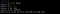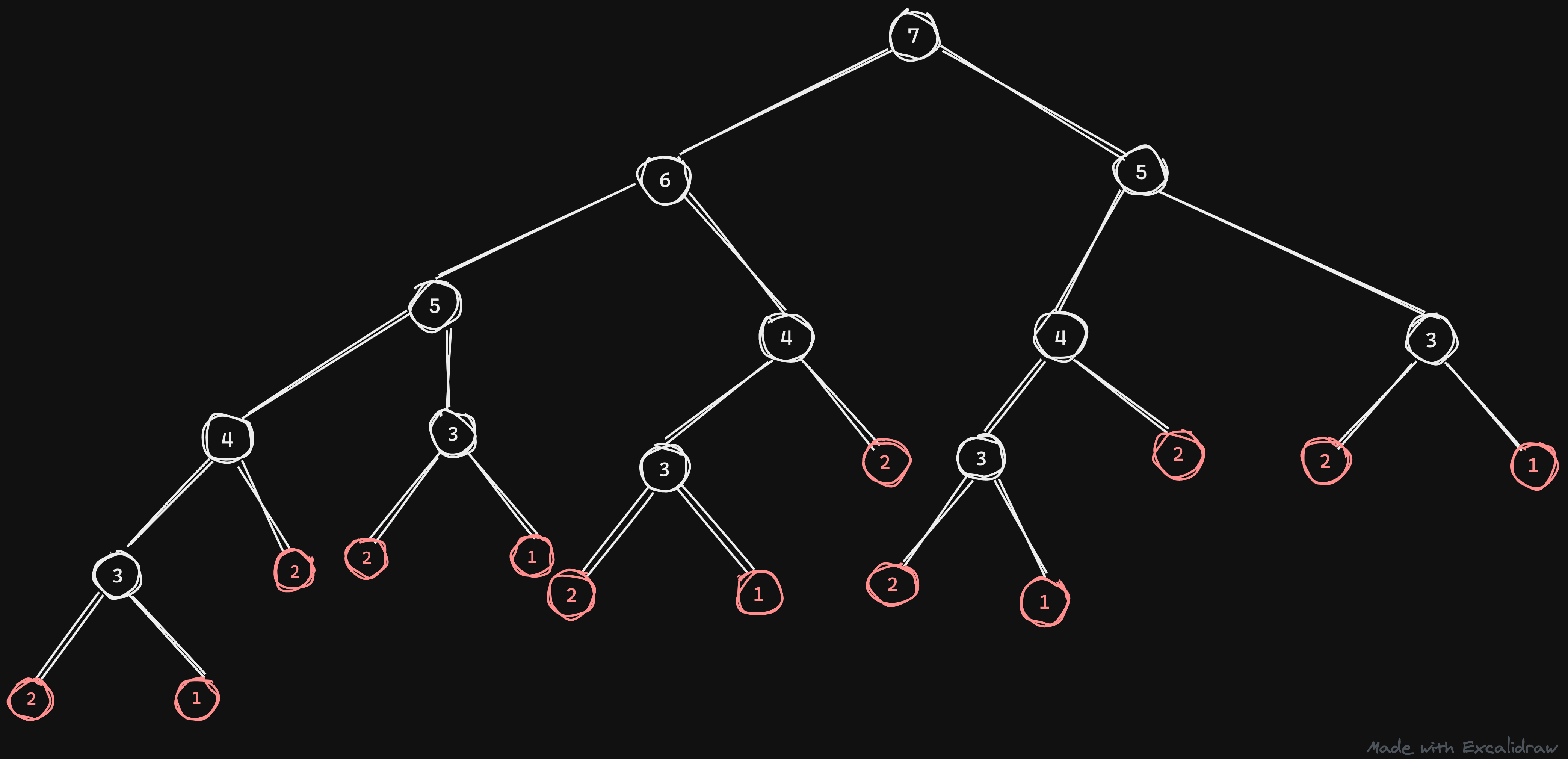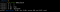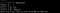Photo by Roberto Carlos Roman Don on Unsplash

# A faster way to find the nth Fibonacci number from it’s series

am assuming you all might know what a Fibonacci sequence is, For those who don’t know, it’s the sequence of numbers such that each number is the sum of the two preceding ones, starting from 0 and 1. For example please refer to the following sequence.

`0, 1, 1, 2, 3, 5, 8, 13, 21, 34, 55, 89, 144, 233, 377, 610, 987, 1597, 2584, 4181, 6765`

## Why Fibonacci?

The question of finding a specific number from the series is what you could expect is a basic coding interview. Anyone from a coding background would know how to do this. Generally, the Fibonacci series is used when teaching recursion and it’s a great example to show how we could achieve recursion.

You might think why is it such a big deal that it is something taught from the college and why should we think of an alternative path to solve this problem. Have you notice the time taken to get a specific Fibonacci number from the series whose index is 100 or above. It will take a lot of time to calculate the result. This is because the time complexity of the problem is `O(2^n)` it means it will take an exponential time when finding a number`n` if it increases.

## Recursion

The traditional method used to find the Fibonacci series is by using the following steps.

1. Check if the number `n` is less than or equal to 2 and return 1 if it is true else continue to the next step which is recursion.
2. Find the Fibonacci number at the index `n-1`and add it to the Fibonacci number at `n-2`

The following illustrates the time required to find the different Fibonacci numbers using the recursion method.Find nth Fibonacci number using the function fib

Notice the time taken it takes to find the 40th Fibonacci number. It takes more time when the number increases.Time is taken for the current execution

This method is the easiest when it comes to faster implementation. The time complexity for the given program is `O(n^2)`.The graph illustration of the recursive execution

The above graph shows how the recursive method executes. The depth of the tree is `n` and on each level, it will have 2 sub-nodes which are split into two, and so on. so the execution goes like `1*2*2*2*...` Thus we conclude having `2^n` steps, hence we have the time complexity `O(2^n)`.

What about the space complexity. You might think the same way what might happen for time complexity but actually, the space complexity is less which is `O(n)`. Take the leftmost bottom side of the graph it will go down the nodes to node number `2` and goes back to `3` and goes to `1` Thus on execution, the maximum stack depth is `n` and it doesn’t exceed that value.

## Memoization

So what is memoization? memoization or memoisation is an optimization technique used primarily to speed up computer programs by storing the results of expensive function calls and returning the cached result when the same inputs occur again.

The memoization does the following.

1. checks if the number is present in the memory dictionary and if it is there it will return the value.
2. It checks if the number is less than or equal to 2 and returns 1 if true.
3. Then after that the recursive call with the `n-1` and `n-2` is called but the change is that the memorization dict is also passed.The execution of the memoization

Notice the function which was slower for 40 now executes faster. This is due to the recall happening within the function. What happens is that on execution it will check for the key in the memo dict and if not present then it tries to save the function results in the memo dict after getting the result.The time required to run the new memoization method.

For example, take the graph which is shown before, you can see that certain functions are executed multiple times like node 3 is present under node 5 and node 6. Hence the function with 3 is executed multiple times. So to prevent this we used the memoization method that saves the time required to re-execute the function.

The complexity of the current method is `O(n)`. Take the graph of the current method that looks like this. The depth of the graph is `n` and we will get 2n executions on each node taking the time complexity for the current graph we will have `O(n)`.

Thus the time complexity of this method is the same as the depth of the graph which is `O(n)`. The space complexity is also the same which is `O(n)`.

## Using lru_cache

So what is `lru_cache`? `lru_cache` Is a decorator to wrap a function with a memoizing callable that saves up to the `maxsize` most recent calls. It can save time when an expensive or I/O bound function is periodically called with the same arguments.

If `maxsize` is set to `None`, the LRU feature is disabled and the cache can grow without bound.The execution using lru_cache

This has the same time complexity as that of the memoization method. The main difference is that we are manually doing the memoization in the previous method but in the current method it is automatically taken care of, the `lru_cache` decorator is saving the function result by itself. `lru_cache` has the option to set the size of the maximum call that it should memorize.The time required to execute this method.

## Conclusion

To summarize we learned how to find n-th Fibonacci using a faster method called memoization and also using `lru_cache`. This doesn’t mean you have to use this method. You could use any method that you find suitable and try out different steps other than this.

## Credits

Huge credit goes to Alvin Zablan from Coderbyte who has shared this amazing explanation via his course which was uploaded to youtube on the freeCodeCamp.org channel. There are other problems related to memoization explained in this video do please check this out.

Also, the StackOverflow thread which gives a clear explanation of the same.

Please provide your feedback and suggestions.

Linkedin: linkedin.com/in/joel-hanson/

Portfolio: joel-hanson.github.io/

Github: github.com/Joel-hanson

Twitter: twitter.com/Joelhanson25

## More from Joel Hanson

Works at @impressAI